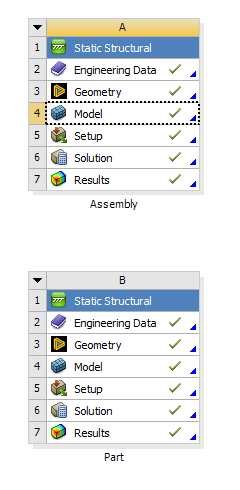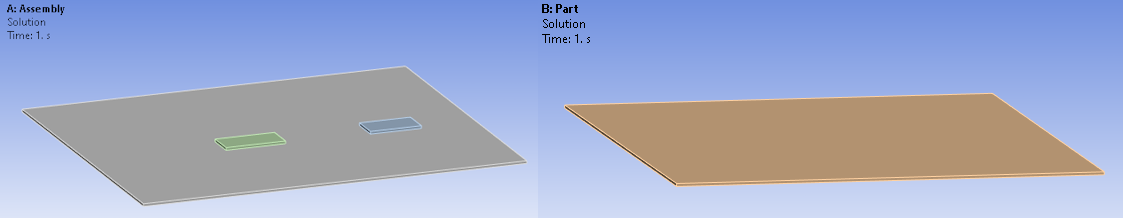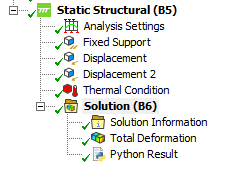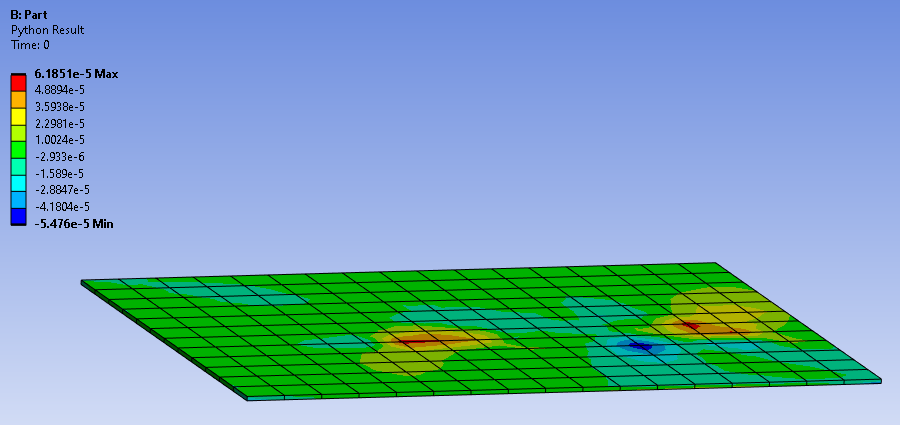# How to map results data into different geometries?

Here's an example which maps the results from an assembly to a specific part of the assembly. The result calculated is the max principal elastic strain. Following the script from the mapping data post, the updated script calculates the difference between the elastic strains of the part and the elastic strains of the assembly. Also a scale factor is added in lines 36-39.Add a Python result object in SolutionCopy paste the following script and modify the path

```def post_started(sender, analysis):# Do not edit this line
define_dpf_workflow(analysis)

def define_dpf_workflow(analysis):

import os
import mech_dpf
import Ans.DataProcessing as dpf
mech_dpf.setExtAPI(ExtAPI)

path_to_other_rst = os.path.join(r'C:\Users\Test','file.rst') # MODIFY THIS TO YOUR PATH
analysis = ExtAPI.DataModel.Project.Model.Analyses

dataSource_1 = dpf.DataSources(analysis.ResultFileName)
dataSource_2 = dpf.DataSources(path_to_other_rst)

model_1 = dpf.Model(dataSource_1)
mesh_1 = model_1.Mesh
model_2 = dpf.Model(dataSource_2)
mesh_2 = model_2.Mesh

seqv_1 = dpf.operators.result.elastic_strain_principal_1()
seqv_1.inputs.data_sources.Connect(dataSource_1)
seqv_2 = dpf.operators.result.elastic_strain_principal_1()
seqv_2.inputs.data_sources.Connect(dataSource_2)

mapping_op = dpf.operators.mapping.on_coordinates()
mapping_op.inputs.coordinates.Connect(mesh_1.CoordinatesField)
mapping_op.inputs.fields_container.Connect(seqv_2.outputs.fields_container)
mapping_op.inputs.mesh.Connect(mesh_1)

minus_fc = dpf.operators.math.minus_fc()
minus_fc.inputs.field_or_fields_container_A.Connect(seqv_1.outputs.fields_container)
minus_fc.inputs.field_or_fields_container_B.Connect(mapping_op.outputs.fields_container.GetData())

scale_field = dpf.operators.math.scale()
scaleValue = 1
scale_field.inputs.field.Connect(minus_fc)
scale_field.inputs.ponderation.Connect(scaleValue)

output = dpf.operators.utility.forward()
output.inputs.any.Connect(scale_field)

dpf_workflow = dpf.Workflow()